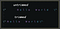# Implementation of the C Function strtrim()Notice the difference between the strings. Some extra spaces in there, right?

First, let’s take a look at the man page of this function. We can use the FreeBSD version of this function.

`strtrim removes all whitespace characters from the beginning and the end of a string.`

In other words, it will remove all the unnecessary white that you might have in a string, for instance:

`char *str = "   Hello world! "             ^^^            ^`

Now that we know what it to do, let’s divide this implementation into small steps.

1. Get the position within the string, where there is no white space at the beginning of it. In the case above, it would be `index = 3`, because `str == 'H'`
`/*** Auxiliary function to skip white spaces*/int is_white_space(char c) {    return (c == ' ' || c == '\t' || c == '\n');}/*** Iterate through the whitespaces at the beginning of the string*/int get_first_position(char const *str) {    int i = 0;    while (is_white_space(str[i])) {        i += 1;    }    return (i);}`

2. Now let’s find the last valid position in the string.

`str == '!'`

`/*** Get the length of a string*/int get_str_len(char const *str) {    int len = 0;    while (str[len] != '\0') {        len += 1;    }    return (len);}/*** Find the last position in a string that is not a white space*/int get_last_position(char const *str) {    int i = get_str_len(str) - 1;    while (is_white_space(str[i])) {        i -= 1;    }    return (i);}`

3. And then let’s copy this into a new array of character with the correct size, so we can have the length of the `"Hello World!"` string, without any white space at the beginning and or end of this string.

`/*** Returns the correct length of a trimmed string*/int get_trim_len(char const *str) {    return (get_last_position(str) - get_first_position(str));}`

4. And now we can copy the correct characters to our `trim` string.

`/*** Allocates a new string with removed whitespace characters from the beginning of the source string `str`*/char *strtrim(char const *str) {// Variables declaration    char *trim = NULL;    int i, len, start, end;// Check if the string exists    if (str != NULL) {        i = 0;        len = get_trim_len(str) + 1;        trim = (char *)malloc(len);        start = get_first_position(str);// Copy content to trim string        while (i < len) {            trim[i] = str[start];            i += 1;            start += 1;        }// Null terminate the trimmed string.        trim[i] = '\0';    }    return (trim);}`

And now, we can create a new c file called `trim.c` and compile the `strtrim()` function implementation with a `main()` function.

`#include <stdio.h>#include <stdlib.h>/*** Auxiliary function to skip white spaces*/int is_white_space(char c) {    return (c == ' ' || c == '\t' || c == '\n');}/*** Iterate through the white spaces at the beginning of the string*/int get_first_position(char const *str) {    int i = 0;    while (is_white_space(str[i])) {        i += 1;    }    return (i);}/*** Get the length of a string*/int get_str_len(char const *str) {    int len = 0;    while (str[len] != '\0') {        len += 1;    }    return (len);}/*** Find the last position in a string that is not a white space*/int get_last_position(char const *str) {    int i = get_str_len(str) - 1;    while (is_white_space(str[i])) {        i -= 1;    }    return (i);}/*** Returns the correct length of a trimmed string*/int get_trim_len(char const *str) {    return (get_last_position(str) - get_first_position(str));}/*** Allocates a new string with removed whitespace characters from the beginning of the source string `str`*/char *strtrim(char const *str) {    char *trim = NULL;    int i, len, start, end;    if (str != NULL) {        i = 0;        len = get_trim_len(str) + 1;        trim = (char *)malloc(len);        start = get_first_position(str);        while (i < len) {            trim[i] = str[start];            i += 1;            start += 1;        }        trim[i] = '\0';    }    return (trim);}/*** Let's rock and roll*/int main(int argc, char const *argv[]) {    if (argc > 1) {        for (int i = 1; i < argc; i += 1) {            printf("[%d] \"%s\"\n", i, strtrim(argv[i]));        }    }    return (0);}`

Let’s test it out!

`> gcc trim.c> ./a.out "  Neque porro quisquam" "      est qui dolorem ipsum quia     " "dolor sit amet" " consectetur" "adipisci " "   velit.. .    "`

Resulting in the following output…

` "Neque porro quisquam" "est qui dolorem ipsum quia" "dolor sit amet" "consectetur" "adipisci" "velit.. ."`

And so, we are done with our strtim() implementation. Hope this has been a good read to get you started on doing implementations of other functions!

Peace out.